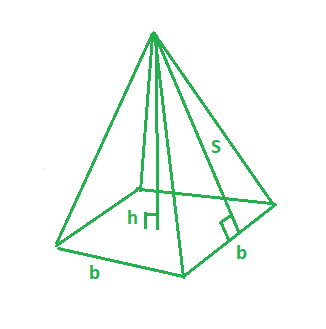# Year 9 Maths Chapter 4 – Linear Relationships

You will want to refer to Year 8 Maths Chapter 3 for basic measurement. This section is more advanced, and omits basic concepts.

## New formulae

• Surface area of a rectangular prism: `A = 2(xy + xz + yz)` where `x`, `y` and `z` are length, breadth and height.
• Volume of a cylinder: `A = 𝜋r²`
• Surface area of a cylinder: `A = 2𝜋r² + 2𝜋rh` where `2𝜋r²` is the 2 circular ends and `2𝜋rh` is the curved area. If you wanted an open cylinder with only one end closed you would remove the 2 in front of the first term.
• Volume of a sphere: `(4/3)𝜋r³`
• Surface area of a sphere: `4𝜋r²`
• Surface area of a square based pyramid: `A = b² + 2bs` where `b` is one base side and `s` is the slant height.

Note that for square based pyramids, you might need to use Pythag to find the slant height. This does not equal the height of the pyramid.In the above image, `h` is the height and `s` is the slant height. By using Pythag you can solve for `s` by using the equation: `h² + (b/2)² = s²`.

## Formulae to revise

#### Circles

• Circumference of a circle: `C = 2𝜋r` or `C = 𝜋d`
• Area of a circle: `A = 𝜋r²`
• `𝜋 = ~22/7` or `𝜋 = 3.14 (2dp)`
• A sector is a portion of a circle.
• Perimeter of a sector: `P = 2r + θ/360 x 2𝜋r`
• Area of a sector: `A = 𝜋r² x θ/360`

#### Rhombus, Kite, Trapezium and Parallelogram

• Rhombus:
• `pq/2` where `p` and `q` are diagonals
• `lb` where `l` is length and `b` is height
• Kite:
• `pq/2` where `p` and `q` are diagonals
• `xy/2` where `x` is width and `y` is height
• Trapezium: `a+b/2 x h` where `a` and `b` are the parallel sides and `h` is the height
• Parallelogram: `lb` where `l` is length and `b` is height

Please tell me you know what the area of squares, rectangles and triangles are. Marcus probably doesn’t.

#### Composite shapes

Shapes that are made up of more than one basic shape. To solve for the area or perimeter of composite shapes, you might need to use addition or subtraction. I know how hard that is for you, Marcus.

Copyright © 2017-2020 aidswidjaja and other contributors. CC BY-SA 4.0 Australia unless otherwise stated.